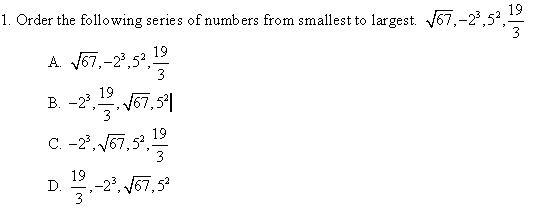Showing posts with label Maths. Show all posts
Showing posts with label Maths. Show all posts

## Saturday, November 13, 2010

### According to the rain gauge, how much rain has fallen?

14.  The Candy Shoppe sells a box of mixed chocolates.  The box of chocolates contains 12 ounces of chocolate covered caramels, 14 ounces of chocolate covered cherries, 6 ounces of chocolate covered raisons. How many pounds are in the box of chocolates?
A.  3.2 pounds
B.  2 pounds
C.  3.6 pounds
D.  3 pounds

15.  According to the rain gauge, how much rain has fallen?

A. 2.7 inches
B. 2.2 inches
C. 2.5 inches
D. 2.4 inches

16. Early civilizations used a cubit as a unit of measure.  A cubit is the length from the middle finger to the elbow.  Estimate the average height of a man in cubits
A.  6 cubits
B.  4 cubits
C.  2 cubits
D.  10 cubits

17.  Jay traveled from Miami to Jacksonville, a distance of 320 miles.  He left Miami at 8:00 A.M., stopped for lunch for 30 minutes, and stopped for two 15 minute breaks at rest areas.  He arrived in Jacksonville at 2:15 P.M.  What was his average rate of speed during his travel time?

A.   51.2 mph        B.   62.1 mph        C.   53.3 mph        D.   60.9 mph

18.  Jackie is building a doll house with a scale of 1 inch equals 12 inches.  She wants to put a sofa in the doll house living room.  If a full size sofa is 72 inches, how long will the doll house sofa be?

A.   7.2 inches
B.   6 inches
C.   5 inches
D.   4 inches

### You purchase a house for \$124,000 and the next year sell it for \$150,000 . What would be the percent of increase or decrease?

7.    What is the correct order of operations for this problem?
A.        B.       C.       D.

8. You purchase a house for \$124,000 and the next year sell it for \$150,000 . What would be the percent of increase or decrease?

A. 21% increase     B.  21% decrease      C.  17% increase    D.  17% decrease

Section 2 - Measurement

9. A swimming pool is 15 by 30 feet and a consistent 5 feet deep.  You are painting the walls and floor of the pool.  If a gallon can of paint covers 250 square feet, how many gallon cans would you need to buy?
A. 2 gallons
B. 3 gallons
C. 4 gallons
D. 5 gallons

10.  You need to fill the pool with water.  If 1 cubic foot equals 7.48 gallons of water, how many gallons will it take to fill the pool in the previous problem (15 feet by 30 feet by 5 feet)?
A. 374 gallons
B. 16830 gallons
C. 300.8 gallons
D. 2250 gallons

11. Pencils sell for 98 cents per dozen.  How much more per pencil does it cost to buy 5 for 50 cents?
A.  8.17 cents         B.  10 cents    C.  1.83 cents        D.  2 cents

12. The testing center starts the test at 8:40 A.M.  If you have 90 minutes to complete the test, when does the test end?
A.  9:30 A.M.
B.  10:10 A.M.
C.  10:40 A.M.
D.  10:30 A.M.

13.  The distance between Brookville and Center City is 17.25 cm. on the map.  If 1 cm is equal to 20 miles, how far is it between the two cities?
A.  37.25 miles
B.  340 miles
C.  345 miles
D.  350 miles

### Practice Test for the General Knowledge Math Test

Practice Test for the General Knowledge Math Test

Directions: Read each item and select the best response.

Section 1 – Number Sense2. Order the following series of numbers from smallest to largest.
5.3x10-3,  1.2x102,  2.7, 2.07

A.  5.3x10-3,  1.2x102,  2.7, 2.07

B.  1.2x102,  2.7, 2.07,  5.3x10-3

C.  5.3x10-3, 2.07, 2.7, 1.2x102,

D.  1.2x102, 2.07,  2.7, 5.3x10-3

3. Simplify    3(2+6)26
A.  3                B.  9                 C. 32               D. 60

4. Simplify    5 + 6 2 -1
A.  7                B.  8                 C. 4.5              D. 11

5.  Jack is taking medications for a recent illness.  Every 6 hours he takes an antibiotic, every 4 hours he takes a pain reliever, and every 3 hours he drinks a glass of water.  If he starts this regime at 10 am, at what time will he be taking both medicines and a glass of water?
A. 12:00 noon              B.  4:00 P.M.               C.  6:00 P.M.               D. 10:00P.M.

6. Three lengths of 2.25 feet each are cut from a board 12 feet long.  How long is the remaining piece of wood?
A. 9.75 feet                  B.  9 feet          C.  5.25 feet                 D.  1.05 feet